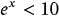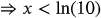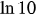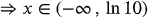1
Chapter
1.6
Section
71
Page
53-a
Problem

# Problem 53-a

## Chapter 1 | Section 1.6 | Page 71Textbook ExpertVerified Tutor
14 Oct

#### Given information

Given equation is.

#### Step-by-step explanation

Step 1.

On simplification, we getTherefore the solutions of the given inequality is the set of all real numbers less than. That is,